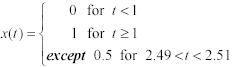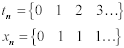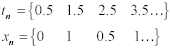# Effect of Inadequate Sampling Rate

Aliasing is the word for the class of problems that result from an inadequate sampling rate. In old Western movies you will sometimes see an extreme form of aliasing cause wagon wheels to turn backwards . This happens because the movie camera takes discrete samples of the wheels at an inadequate rate. If the wheels turn almost one full spoke between each sample, it creates the illusion that the wheels are precessing slowly backwards in successive frames .

In simulations of high-speed digital systems an inadequate sampling rate causes a waveform to "wiggle around" as a function of precisely where it is sampled. For example, suppose you are sampling a continuous-time function with a step at time 1 and a small glitch near time 2.50.

Equation 4.14Let the sampling interval D T be 1 second. As the samples t n progress through the continuous-time waveform, you get different results depending on where the samples start. For example, samples starting at t = 0 look like this:

Equation 4.15A train of samples taken starting near t = 0.5, however, would produce this totally different result:

Equation 4.16Which is correct? The answer, of course, is neither . The waveform defined in [4.14] contains transitions far too quick to be sampled at such a pedestrian rate as 1 sample per second.

Figure 4.3 illustrates the kind of aliasing you are likely to encounter with the frequency-domain simulation if your sampling rate is too slow. This figure shows two rising edges. One is a linear ramp, the other a Gaussian rising waveform. The rise time in both cases is set to 50 ps. The sample interval is 12.5 ps, providing four samples during each rising edge.

Figure 4.3. The Gaussian rising edge displays less wiggling (Gibbs phenomena) than the linear-ramp edge.Four frequency-domain simulations are shown. In each simulation the time-domain waveform is sampled and converted to the frequency-domain. The frequency-domain vector is then delayed (using the frequency-domain delay operator) by 1/4, 1/2;, and 3/4; of a sample time. The inverse FFT is then computed for each case, normalized, and the time-domain displays are then successively offset back in time to overlay the original signal. If the sampling rate were infinite, the time-domain shifting would precisely cancel the frequency-domain delay operator, and the waveforms would overlay perfectly . As you can see, they do not.

The linear-ramp edge shows a classic pattern of frequency-domain truncation (Gibbs phenomena) . The limited sampling rate imposes a maximum value on w k , a value which is apparently too small to capture all of the significant high-frequency information present within the signal. The missing high-frequency content induces extraneous wiggles in the signal before and after the rising edge indicating a sensitivity to precisely how the time-domain samples align with the waveform. If you've ever used a scope with an inadequately fast sampling rate, you have probably seen a similar effect ”the sampled waveform appears to "jiggle" on the screen even when you know it contains no jitter.

The figure also shows a Gaussian-rising edge simulated using the same oversampling rate and the same delay procedures. The Gaussian rising edge clearly displays less Gibbs phenomena than the linear-ramp edge. With Gaussian edges an oversampling of 4x the risetime generates a sufficiently dense grid to produce a good representation of the underlying continuous-time signal regardless of the sampling phase alignment.

An FFT theorist would say that the frequency content of the Gaussian-filtered edge is sufficiently suppressed at the Nyquist rate (0.5/ D T Hz) to avoid aliasing.

POINT TO REMEMBER

• In simulations of high-speed digital systems an inadequate sampling rate causes a waveform to "wiggle around" as a function of precisely where it is sampled.High-Speed Signal Propagation[c] Advanced Black Magic
ISBN: 013084408X
EAN: N/A
Year: 2005
Pages: 163